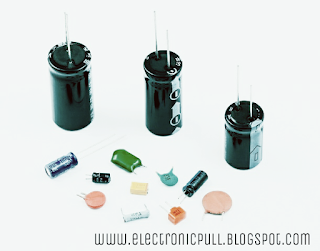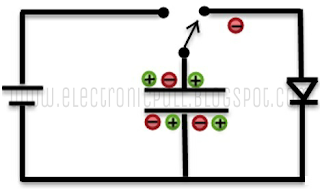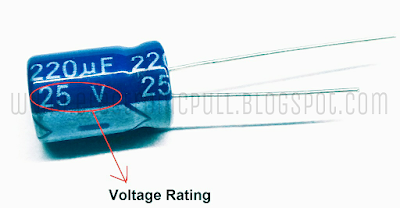# Introduction to Capacitor-Titik Letak-Titik Letak

Definition:
Capacitor is an electronic device which stores the charge in the form of an electric field, also known as condenser. Capacitor is also the passive component like resistor. Generally, it is used to store the charge.Different types of cccccapacitors
Capacitor symbols:Capacitor's symbols
Importance:

Capacitors are important because:
• They stores the energy and can release this energy when required to the circuit.
• They are used to couple one part of the circuit to the other by allowing only DC to flow and prevent AC to flow.
• Capacitors are also used to amplify the frequency when required by the circuit.
• In power applications, when the capacitor is applied with an AC input then it increases the circuit's pay load power due to which the current leads the voltage.
• Capacitor allows high frequencies to pass and it is used as a filters to filter low or high frequencies.
• Capacitors can also be used to maximize or minimize the circuit's impedance at corresponding frequency by using it as a filter.

Construction:

It has two metal plates parallel to each other, which are not connected. These two plates are separated by non-conducting material (insulating material) which is commonly known as Dielectric medium.
There are many capacitors that are different in size and dimension but all of them are are used in storing the electrical charge in them.
The capacitors are present in different shapes like rectangle, square, circular, cylindrical shapes. An ideal capacitor, unlike resistor, does not dissipate energy.

Dielectric:

Dielectric is the material between the two plates. Dielectric is of different type of non-conducting material such as ceramic, mica, plastic or some type of a liquid gel and waxed paper. It plays an important in finding the value of capacitance of the capacitor. As, the insulated material or dielectric is placed between the plates in the centre of the capacitor then its value increases.
The value of dielectric constants are different for dielectric materials whatever, the value is greater than 1.
The following below table gives the value of dielectric constant for different materials.

Dielectric's types:

The two types of dielectrics are followings:
Polar dielectrics: These type of dielectrics have permanent movement.
Non Polar dielectrics: These types of dielectrics have temporary dielectric movement. They can be induced with the dipole moments by placing them in an electric field.
The insulated materials which are used in capacitors as a dielectric are as following:
• Ceramic
• Paper
• Plastic film
• Mica
• Glass
• Tantalum Pentoxide
• Aluminium Oxide
• Niobium Pentoxide

Complex Permittivity:

It is the product of the relative permittivity of free space (εo) with the relative permittivity (εr) of the dielectric material, which is called complex permittivity of the dielectric material. The formula of the complex permittivity is given below,

ε = ε0 * εr

The value of the complex permittivity for the capacitor is equal to the relative permittivity, because the relative permittivity of free space is equal to ‘1’. The value of complex permittivity differs from one material to another. Some of the values of complex permittivity (ε) for different dielectric materials are'
Pure Vacuum = 1.0000, Air = 1.0005, Mica = 5 to 7, Paper = 2.5 to 3.5, Wood = 3 to 8, Glass = 3 to 10 and Metal Oxide Powders = 6 to 20 and etc.

Working:

As capacitor consists of two conductors, separated by a insulated material, when there is any voltage or potential difference moves between the two conductors then the electric potential is developed then this causes the capacitor to both charge and discharge.
In the practical way, when the capacitor is connected to a a DC source, then the  current starts to flow through the circuit. Thus, -ve charge is stored on one plate and +ve charge is stored on the other plate. This whole process continuous until the voltage of capacitor reaches the supply voltage. When the voltage of the capacitor is equal to the input voltage, then the capacitor tends to stop charging further even when the battery is connected to the circuit. After this, when the battery is removed from the circuit the the two plates will be accumulated with +ve and -ve charges. Thus, the capacitor stores the charge. But, when the input supply voltage is of an AC source then, it both charges and discharges continuously.

Example
Below diagram shows the working of capacitor.Working Figure (1)Working Figure (2)

Capacitance:

Capacitance is the factor of the capacitor that describes the maximum amount of electrical charge that can be stored in it. The value of capacitance carries according to the shape of the capacitor. Its value can be calculated by determining the characteristics about conducting material and dielectric material.
Capacitance can be defined as the ratio of charge (Q) on the plates to the potential difference (V) between them.

C =Q/V,

The unit of capacitance is Farads (F).
The capacitance is inversely proportional to the voltage (V) and directly proportional to the charge (Q). Where, the capacitance increases by increasing the no. of plates, which keeps the same size of the capacitor.

Standard units of capacitance:

The capacitance can be expressed in Farads (F) and can also be expressed such as as micro farads(uF) , nano farads(nF) and pico farads(PF).
Where as,
• 1 mF (milli farad) = 10−3 F = 1000 μF = 1000000 nF
• 1 μF (microfarad) =10−6 F = 1000 nF = 1000000 pF
• 1 nF (nano farad) = 10−9 F = 1000 pF
• 1 pF (picofarad) = 10−12 F

Voltage Rating of a Capacitor:

It is the maximum voltage by which a capacitor charges to a maximum value also called as working voltage (WV) or DC working voltage (DC-WV). The figure below expresses the voltage rating of the capacitor.
If the large voltage is applied to the capacitor then it damages the capacitor by developing an arc between the plates due to the break down of dielectric.
The voltage rating plays important role, while designing the circuits with capacitors, and the capacitor's voltage rating should be greater than the voltage used in the circuit e.g. if the circuit operates at 12V then you should choose a capacitor with voltage rating of 12V or above. This voltage rating depends on the factors like thickness of dielectric, material used as dielectric between the plates and on the circuit.Voltage Rating of Capacitor